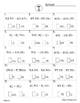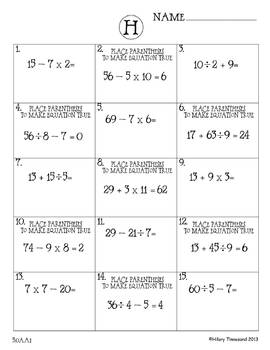30 Common Core ** 5th Grade** Math Timed Tests (assessments or test prep)Subject
Resource Type
Common Core State Standards
Product Rating
File Type

PDF (Acrobat) Document File

Be sure that you have an application to open this file type before downloading and/or purchasing.

124 MB|65 pages
Share
Product Description
30 Math Timed Test designed specifically to meet the 5th grade Common Core Standards. Use daily for the entire school year. Designed to progress through the Common Core Standards. Quick daily timed tests are also great for homework. Build student confidence and math skills easily as students are self-motivated to progress to the next level. There is so much flexibility with this system you can truly tailor it to fit your needs. You can use as practice, test prep or standards assessment.

Personally I used these as daily timed tests and feel like they contributed greatly to the students understanding of math and confidence. My school is 94% free and reduced lunch, 80% Hispanic and 71% ELL (half my class had not yet passed the state English language proficiency test). On our state test last year our school wide 3rd grade performance in math was a 46% passing rate my class pass 65% in math. I think the timed test and math notebook graphic organizers (in separate listing) were key to our success.

5.NBT.A.1 Expanded and standard form
5.NBT.A.2 Multiplying and dividing by the power of 10
5.NBT.A.3 Read, write and compare decimals to the 1000’s
5.NBT.A.4 Rounding then add / sub decimals
5.NBT.B.5 Multiply multi-digit numbers
5.NBT.B.6 Dividing with remainders
5.NBT.B.7 Add, subtract, multiply, and divide decimals
5.OA.A.1 Using parentheses in expressions
5.OA.A.2 Write numerical expressions
5.OA.B.3 Numerical patterns
5.NF.A.1 Adding and subtracting fractions
5.NF.A.1 Reducing fractions to simplest form
5.NF.A.1 Adding and subtracting fractions w/ unlike denominators
5.NF.A.1 Determine missing addends in fractions
5.NF.B.3 Interpret fractions from visual models
5.NF.B.4 Multiply a fraction by a whole number
5.NF.B.6 Multiplying fractions and mixed numbers
5.NF.B.7 Division with fractions and whole numbers
5.MD.A.1 Conversions of units
5.MD.A.1 Conversions of units with addition and subtraction
5.MD.B.2 Determine line plot data
5.MD.C.3 Find volume of solid figures
5.MD.C.4 Volume measurement by cubic units
5.MD.C.5a Determine cubic volume
5.MD.C.5b Determine volume of irregular shapes
5.G.A.1 Plot coordinates
5.G.A.2 Shifting plot coordinates
5.G.A.3 Understanding 2 dimensional shape attributes
5.G.A.4 Classify 2 dimensional figures
5.G.A.4 Understanding open and closed shapes

* Also includes answer sheets for each test, teacher record sheet, and individual student progress charts.
Total Pages
65 pages
N/A
Teaching Duration
N/A
Report this Resource to TpT
Reported resources will be reviewed by our team. Report this resource to let us know if this resource violates TpT’s content guidelines.